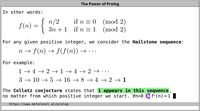# Basic Concepts

We introduce and define the most basic concepts of Prolog.

## Terms

In Prolog, all data—including Prolog programs—are represented by Prolog terms.

## Programs

A Prolog program is a set of predicates.

Predicates define relations between their arguments. Logically, a Prolog program states what holds.

There are a few conventions for writing Prolog programs, and different ways of reading them.

### Predicates

Each predicate has a name, and zero or more arguments. The predicate name is a Prolog atom. Each argument is an arbitrary Prolog term.

A predicate with name Pred and N arguments is denoted by Pred/N, which is called a predicate indicator. N is called the arity of the predicate.

 Video: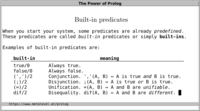A predicate is defined by a collection of clauses.

A clause is either a rule or a fact. The clauses that constitute a predicate denote logical alternatives: If any clause is true, then the whole predicate is true.

 Video: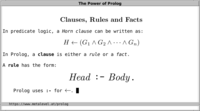### Rules

A Prolog rule has the form:
```Head :- Body.
```
The notation of the head of a rule depends on the number of arguments:
• If the predicate has zero arguments, then the head consists only of the predicate name.
• If a predicate called Name has a positive number N of arguments, then the head is written as: Name(Arg1, Arg2, ..., ArgN).
The body of each rule is a Prolog goal.

A goal is a Prolog term that denotes a predicate and its arguments.

A rule is called recursive if one of its goals refers to the predicate that the rule is defining.

### Facts

A fact is written as:
```Head.
```
This is equivalent to the rule:
```Head :- true.
```
Logically, this means that the rule always holds, because the built-in predicate true/0 is always true.

## Toplevel

The Prolog toplevel is the main way in which we run Prolog programs.

 Video: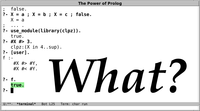We invoke a Prolog predicate by posting a query on the toplevel. A query is an arbitrary Prolog goal. In a query, variables are existentially quantified. We can thus read a query as: "Are there any cases for which the given predicate holds?"

 Video: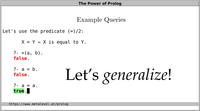In response to a query, the toplevel reports an answer. The answer is a Prolog goal that is declaratively equivalent to the query.

Every predicate has an associated most general query, which means that all arguments are fresh variables.

Note that a goal can succeed more than once. Depending on your Prolog implementation, you either press SPACE or ";" to see alternatives.

## Running Prolog code

Running a Prolog program can be regarded as a special case of resolution, which is an algorithm that is rooted in formal logic. Logically, when Prolog answers a query, it tries to find a resolution refutation of the negated query and the set of clauses that constitute the program. When a refutation is found, it means that the query is a logical consequence of the program.

An important step in this process is syntactic unification of terms. Unification is a generalization of pattern matching. When a clause head is chosen for unification with a Prolog goal, then unification applies to the arguments of both.

For this reason, there is no distinction between input and output arguments of pure predicates, and Prolog predicates can often be used in several directions.

If multiple clause heads unify with a goal, then alternatives are tried on backtracking.

Informally, you can think of Prolog's default execution strategy, which is called depth-first search with chronological backtracking, as a generalization of function calls that are available in other languages. The main differences are that: (1) multiple clauses can match and (2) unification works in both directions.

A Prolog program can be interpreted with different execution strategies that can be flexibly selected. SLG resolution is an alternative execution strategy that is available in an increasing number of Prolog systems. The ability to use different execution strategies while keeping the program unchanged is a major attraction of Prolog.

To find mistakes in Prolog programs, it is typically not necessary to trace the actual execution steps. Instead, declarative debugging techniques can be applied to narrow down mistakes by logical reasoning.

## Built-ins

Some predicates are already predefined when you start your Prolog system. These are called built-in predicates or simply built-ins.

For example, the built-in (=)/2 is true iff its arguments unify. The built-in true/0 is always true, and the built-in false/0 is always false.

Many built-ins are only available for convenience, and you could easily define them yourself by the mechanisms explained above. For example, if they were not already defined, you could define the mentioned predicates as:
```T = T.

true.

false :- a = b.
```
However, not all built-ins can be defined in this way.

In addition to (=)/2, true/0 and false/0, the most important built-ins you need to know to write useful and pure Prolog programs are:
• dif/2 is true iff its arguments are different terms
• integer constraints let you reason about arithmetic expressions
• (',')/2 denotes conjunction: (A,B) is true iff both A and B are true
• (';')/2 denotes disjunction: (A;B) is true iff either A or B or both are true.

## Example: Collatz conjecture

We illustrate all these concepts by means of an example:
Take any positive integer N. To get the next integer, do the following:
• If N is even, divide it by 2.
• If N is odd, multiply it by 3 and add 1, obtaining 3×N + 1.
Repeat this indefinitely to obtain the hailstone sequence N0, N1, N2, ...

The Collatz conjecture is that the integer 1 appears in this sequence for all positive initial integers.
We can model the hailstone sequence as follows, using the built-in integer constraint (#=)/2 to denote equality of two integer expressions:
```hailstone(N, N).
hailstone(N0, N) :-
N0 #= 2*N1,
hailstone(N1, N).
hailstone(N0, N) :-
N0 #= 2*_ + 1,
N1 #= 3*N0 + 1,
hailstone(N1, N).
```
The predicate hailstone/2 is defined by three clauses: one fact and two rules. It defines a relation between two arguments. The first argument represents the current element of the sequence. The second argument is used to report solutions. After a query is posted and an answer is reported by the toplevel, the alternatives are tried on backtracking:
```?- hailstone(3, N).
N = 3
;  N = 10
;  N = 5
;  N = 16
;  N = 8
;  N = 4
;  N = 2
;  N = 1
;  N = 4
;  N = 2
;  N = 1
;  ... .
```
This program illustrates that a sequence of actions can be modeled as a relation between successive states. See Thinking in States for more examples.

As is characteristic for pure relations, the predicate can be used in all directions. For example, we can post the most general query:
```?- hailstone(X, Y).
X = Y
;  clpz:(2*Y#=X)
;  clpz:(2*Y#=_A), clpz:(2*_A#=X)
;  clpz:(2*_A#=X), clpz:(2*Y#=_B), clpz:(2*_B#=_A)
;  ... .
```
When studying a Prolog predicate, try the most general query to see what answers look like in general.

We can implement hailstone/2 more efficiently with if_/3.

 Video: